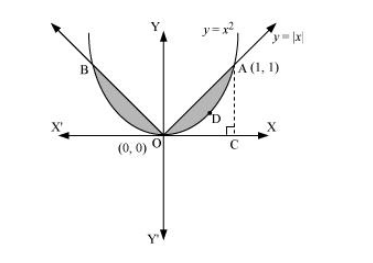# Find the area bounded by curves

Question:

Find the area bounded by curves $\left\{(x, y): y \geq x^{2}\right.$ and $\left.y=|x|\right\}$

Solution:

The area bounded by the curves, $\left\{(x, y): y \geq x^{2}\right.$ and $\left.y=|x|\right\}$, is represented by the shaded region asIt can be observed that the required area is symmetrical about y-axis.

Required area $=2[$ Area $($ OCAO $)-$ Area $($ OCADO $)]$

$=2\left[\int_{0}^{1} x d x-\int_{0}^{1} x^{2} d x\right]$

$=2\left[\left[\frac{x^{2}}{2}\right]_{0}^{1}-\left[\frac{x^{3}}{3}\right]_{0}^{1}\right]$

$=2\left[\frac{1}{2}-\frac{1}{3}\right]$

$=2\left[\frac{1}{6}\right]=\frac{1}{3}$ units